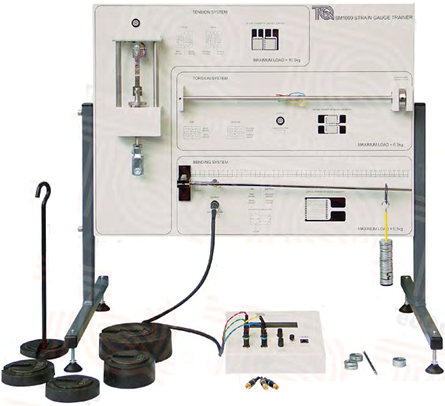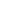### الصفحاتم4 Design مرحلة رابعة

# Strain Gauge Trainer Experiment مختبر التصميم

Experiment No. 7

Name: Strain Gauge Trainer

Aim: To shows and compares how resistance strain gauges work, and how they measure strains in different structures. Later the stresses or the load could be calculated.

Theory:

The compact Strain Gauge Trainer fits on a bench or desktop. It contains everything needed to show how resistance strain gauges work on three different structures. The bending system uses gauges to measure direct tensile and compression strain. The torsion system shows the use of shear / torque strain gauges. The tension system shows the use of two gauges at right angles in a ‘Tee’ rosette. The strain display includes a set of high-accuracy dummy strain gauge resistors (plugs) and controls. These allow the user to connect the strain gauges on the structures as quarter, half or full-bridge networks.

The gauge factor GF is defined as:

Where; 🔺R is the change in resistance caused by strain, RG is the resistance of the undeformed gauge, and Є is strain.

For metallic foil gauges, the gauge factor is usually a little over 2. For a single active gauge and three dummy resistors, the output V from the bridge is:

Where  is the bridge excitation voltage.

In order to measure strain with a bonded resistance strain gage, it must be connected to an electric circuit that is capable of measuring the minute changes in resistance corresponding to strain. Strain gage transducers usually employ four strain gage elements electrically connected to form a Wheatstone bridge circuit. A Wheatstone bridge is a divided bridge circuit used for the measurement of static or dynamic electrical resistance. The output voltage of the Wheatstone bridge is expressed in millivolts output per volt input. The Wheatstone circuit is also well suited for temperature compensation.

Technical Details

 Item Details Fixed Bridge Voltage 5 VDC Nominal Gauge Resistance 350 Ohm Dummy Plugs Resistance 350 Ohm Tension System Specimen E = 207 GPa, ν = 0.30, Cross Section = 10 x 2 mm Bending System Beam E = 207 GPa, ν = 0.30, Cross Section = 20 x 5 mm Torsion System Beam G = 79.6 GPa, ν = 0.30, Dia. = 10 mm, L = 150 mm

Experiments

By the apparatus many experiments can be done through the different bridge connections (quarter, half and full-bridge) like:

1-    Tensile stresses and strains in different materials and comparison of Poisson’s ratio and Young’s modulus

2-   Strains and stresses in a bending system.

3-    Strains and stresses in a torsion system.

A- Tensile Test Procedure:

1-      Make the quarter bridge connection by using one strain gauge for longitudinal strain with dummy strain.

4-      Draw the relation of the calculated stress with the measured strain to get the Young’s modulus.

5-      Repeat procedure 1, 2 & 3 by using one strain gauge for lateral strain measurement.

6-      Draw the relation of the longitudinal and the lateral strains to get the Poisson’s ratio.

7-      Make the half bridge connection by using two strain gauges [ both longitudinal ] with two dummy strain gauges, and repeat step 3.

8-      Make full bridge connection and repeat step 3.

9-      Draw the gotten results from step 7 & 8 with the applied load and get the sensitivity [ slope ] for each case. Then discuss that variation in sensitivity.

Make the below table for each case of the above mentioned steps of quarter, half and full bridge of the strain gauges measurement, then complete the required calculations.

 Measured Dimensions of the specimen = Used Strain Gauge: Quarter Bridge Quarter Bridge Half Bridge Half Bridge Full Bridge Load ( kg ) Force ( N ) Calculated Stress      ( MPa ) Measured Strain  ( μϵ ) Measured Strain  ( μϵ ) Measured Strain  ( μϵ ) Measured Strain  ( μϵ ) Measured Strain  ( μϵ ) Measured Strain  ( μϵ ) 0 0 0 0 0 0 0 0 0 1.0 2.0 3.0 5.5 7.0 8.0

B- Bending Test Procedure:

1-   Make the quarter bridge connection by using one strain gauge for longitudinal strain at the top surface of the cantilever with dummy strain.

2-   Fix the hook load at specified distance from the fixed end and set the strain gauge reading to zero reading.

3-   Increase the load by 10 g load [ 10 pieces of the standard weight ] and record the strain gauge reading for each case till the maximum load.

4-   Make the half bridge connection by using two strain gauges [ both tension ] with two dummy strain gauges, and repeat step 3.

5-   Repeat step 4 by using two strain gauges [ both compression ], and repeat step 3.

6-   Make full bridge connection and repeat step 3.

7-   Make the below table for each case of the above mentioned steps of quarter, half and full bridge of the strain gauges measurement, then complete the required calculations.

 Measured Dimensions of the specimen = Load Position = Used Strain Gauge: Quarter Bridge Quarter Bridge Half Bridge Half Bridge Full Bridge Load (g) Force (N) Bending Moment  ( Nm) Calculated Stress (MPa) Calculated Strain (μϵ) Measured Strain (μϵ) Measured Strain (μϵ) Measured Strain (μϵ) Measured Strain (μϵ) Measured Strain (μϵ) 0 0 0 100 200 300 400 500

8-   Draw the results gained from steps 3 & 4 [measured strain with the calculated stress] to get the modulus of elasticity of the beam and compare with the standard value.

9-   Draw the results gained from steps 4 & 5 [Tension strain with Compression strain] to get the Poissons ratio of the tested beam.

10-  Draw the gotten results from step 3, 4 & 5 with the applied load and get the sensitivity [ slope ] for each case.

C- Torsion Test Procedure:

1-   Make the half bridge connection by using two strain gauges [ both tension ] with two dummy strain  of the torsion rod.

2-   Fit the torque arm at the end of the torsion rod and set the strain gauge reading to zero reading.

3-   Increase the load by 100 g load [ 20 pieces of the standard weight ] and record the strain gauge reading for each case till the maximum load.

4-   Make full bridge connection and repeat step 3.

5-   Make the below table for each case of the above mentioned steps of quarter, half and full bridge of the strain gauges measurement, then complete the required calculations.

 Measured Dimensions of the specimen: Torque Arm Length = Used Strain Gauge: Quarter Bridge Half Bridge Half  Bridge Full  Bridge Load  (g) Force (N) Torque   ( Nm) Calculated Shear Stress (MPa) Calculated Strain (μϵ) Measured Strain (μϵ) Measured Strain (μϵ) Measured Strain (μϵ) 0 0 0 100 200 300 400 500

6-      Draw the gotten results from step 3 & 4  with the applied torque and get the sensitivity [ slope ] for each case.

Discussions

1-   Compare between the calculated modulus of elasticity and Poissons ratio of the specimens that are used in tension and bending test.

2-   Discuss that variation in gained sensitivity from the plotted curves for quarter, half and full bridge for strain connections.

3-   Give practical examples for the using of strain gauges for mechanical measurements like force, moment, torque, pressure or other applications.مهندس ميكانيك
هدفي هو التطوير من ذاتي ثم الاستفادة ثم تطوير غيري